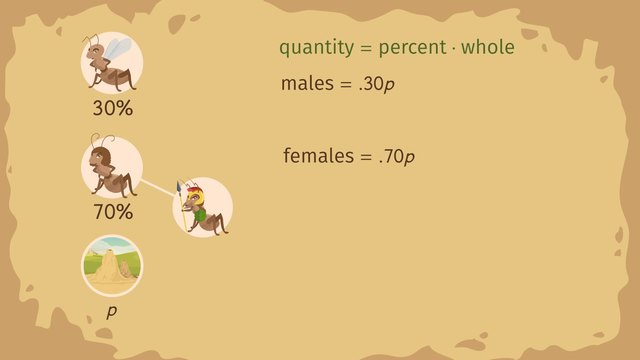# Population ProblemsRating

Ø 5.0 / 1 ratings
The authorsTrentkay

## Basics on the topicPopulation Problems

After this lesson, you will be able to use algebraic expressions to solve problems involving percents related to populations.

The lesson begins by teaching you how to set up an expression with an unknown using algebra. It leads you to the equation, Quantity=Percent x Whole. It concludes with substituting known values in an equation to solve for the unknown.

Learn about population problems involving percents by helping Antonio the anteater determine the percentage of ants he can eat.

This video includes key concepts, notation, and vocabulary such as an unknown variable (the unknown part of the equation that is represented by a letter) and the equation Quantity=Percent x Whole.

Before watching this video, you should already be familiar with using percents to solve problems, solving the equation Quantity=Percent x Whole when given two of the three values, and problems related to percent increase/decrease.

After watching this video, you will be prepared to learn…

Common Core Standard(s) in focus: 7.RP.A.2c, 7.RP.A.3, 7.EE.B.3 A video intended for math students in the 7th grade Recommended for students who are 12 - 13 years old

### TranscriptPopulation Problems

Bienvenidos to beautiful Venezuela where Antonio the anteater is ready for his next meal. Mmm, here's a scrumptious-looking anthill. Antonio loves to eat ants but he's a cautious eater. He doesn't like to eat soldier ants, because they have sharp mandibles! To determine if this anthill has the right percentage of ants for Antonio, we'll have to solve population problems involving percents. To help Antonio, let's first understand how ant colonies are organized. Ant colonies have both male and female ants but only female ants can be soldiers. That means Antonio can safely eat all the male ants in the colony, no problem! In this colony, 30% of the ants are male and 70% are female. But Antonio doesn't know the total ant population in this anthill. Because we have an unknown we can set-up expressions for these situations using algebra. For our unknown ant population we can use the variable 'p.' Recall the equation that says quantity equals percent times whole. Looking at our given information, the quantity we're trying to find is the number of males in the colony. What is the whole in this problem? The WHOLE is the total ant population, 'p'. Finally, the percent of what we're looking for, male ants, is 30 percent. We write the percent as point three zero because to calculate with percents we use the decimal form. Therefore, we can express the number of males ants as point three zero 'p.' Now, what about the female ants? Again, using our equation quantity equals percent times whole we can fill in the information we know. This results in females being expressed as point seven zero 'p' Females definitely make up more of the population but by what percent are there more female ants than male ants? It might be tempting to say 40 percent, because that's 70 percent minus 30 percent. However, we should examine the actual ratio by finding the multiplier from males to females. We can find the percent by isolating it in our equation. Then, wesubstitutein our known values for the female and male parts of the population. Simplifying gives us the ratio seven-thirds or approximately two point three three which multiplied by 100 gives us 233 percent. That means there are 233 percent MORE females than males in this ant colony. Let's figure out how many of those female ants Antonio can consume. To do this we'll need to breakdown the female ant population. Besides the queen, female ants can be workers or soldiers. Worker ants make up 60% of the female population in this anthill and the other 40% are soldiers. Remember, Antonio wants to avoid soldier ants so, how many female ants in this colony can Antonio consume? Well using the same set-up... we can see that the number of workers in the colony is expressed by the percent of females Recalling that the females equal point seven zero of the total population we can substitute that information as well as the percent of workers into our equation. This gives us that the number of worker ants equals point six zero times point seven zero 'p.' Notice that this is actually taking a percent of a percent because the worker ants are a subset of the female ants. Multiplying gives us point four two 'p.' That works out to mean the workers are 42% of the entire ant population. Therefore, in total, Antonio can eat all the male ants, point three zero 'p' and the workers, point four two 'p'. In sum that's point seven two 'p' which means 72 percent of all the ants in the anthill. Great! At least that's more than the total percentage of female ants and should be plenty for hungry Antonio. To review how to calculate population problems involving percents we set up the equation quantity equals percent times whole. Using this equation, if we know two pieces of the information we can always rearrange it to find the third piece. Has this information helped Antonio chose his meal wisely? He's pretty sure he can handle the measly mix of soldier ants today. Lunch time! Uh oh! Looks like an ambush.